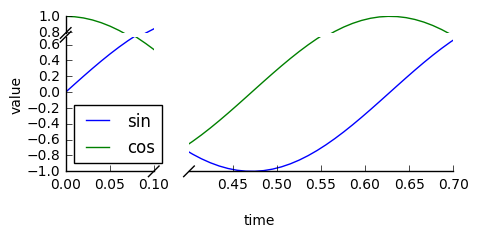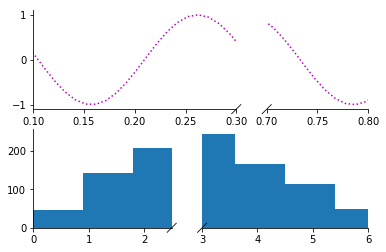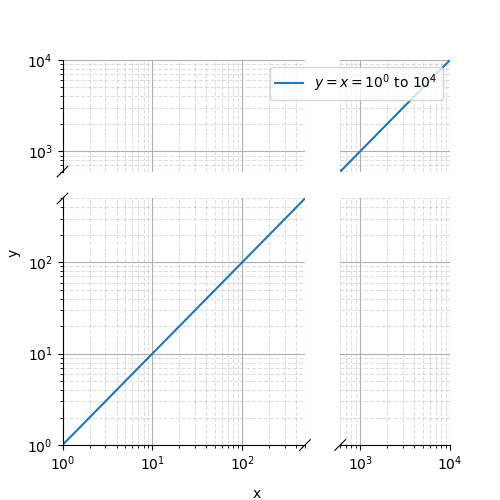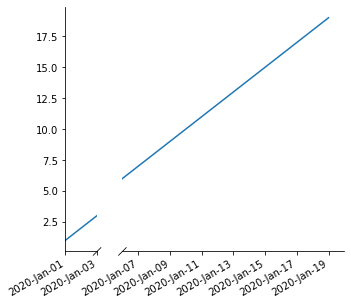Skip to content
{{ message }}

# bendichter / brokenaxes

Create matplotlib plots with broken axes

Switch branches/tags
Could not load branches
Nothing to show

## Latest commit

`3c44dd9`

## Files

Failed to load latest commit information.
Type
Name
Commit time

# brokenaxesbrokenaxes makes matplotlib plots with breaks in the axes for showing data across a discontinuous range.

### Features

• Break x and y axes.
• Supports multiple breaks on a single axis.
• Automatically scales axes according to relative ranges.
• Plot multiple lines.
• Legend with positioning relative to entire broken axes object
• x and y label centered to entire plot
• Make brokenaxes object a subplot itself with `matplotlib.GridSpec.subplot_spec`.
• xlims and ylims may be `datetime.datetime` objects
• Supports log scales.

## Installation

I recommend the Anaconda python distribution and this package is available via pypi:

``````pip install brokenaxes
``````

## Usage

```import matplotlib.pyplot as plt
from brokenaxes import brokenaxes
import numpy as np

fig = plt.figure(figsize=(5, 2))
bax = brokenaxes(xlims=((0, .1), (.4, .7)), ylims=((-1, .7), (.79, 1)), hspace=.05)
x = np.linspace(0, 1, 100)
bax.plot(x, np.sin(10 * x), label='sin')
bax.plot(x, np.cos(10 * x), label='cos')
bax.legend(loc=3)
bax.set_xlabel('time')
bax.set_ylabel('value')```### Create subplots

```from brokenaxes import brokenaxes
from matplotlib.gridspec import GridSpec
import numpy as np

sps1, sps2 = GridSpec(2,1)

bax = brokenaxes(xlims=((.1, .3), (.7, .8)), subplot_spec=sps1)
x = np.linspace(0, 1, 100)
bax.plot(x, np.sin(x*30), ls=':', color='m')

x = np.random.poisson(3, 1000)
bax = brokenaxes(xlims=((0, 2.5), (3, 6)), subplot_spec=sps2)
bax.hist(x, histtype='bar')```### Log scales

```import matplotlib.pyplot as plt
from brokenaxes import brokenaxes
import numpy as np

fig = plt.figure(figsize=(5, 5))
bax = brokenaxes(xlims=((1, 500), (600, 10000)),
ylims=((1, 500), (600, 10000)),
hspace=.15, xscale='log', yscale='log')

x = np.logspace(0.0, 4, 100)
bax.loglog(x, x, label='\$y=x=10^{0}\$ to \$10^{4}\$')

bax.legend(loc='best')
bax.grid(axis='both', which='major', ls='-')
bax.grid(axis='both', which='minor', ls='--', alpha=0.4)
bax.set_xlabel('x')
bax.set_ylabel('y')
plt.show()```### datetime

```import matplotlib.pyplot as plt
from brokenaxes import brokenaxes
import numpy as np
import datetime

fig = plt.figure(figsize=(5, 5))
xx = [datetime.datetime(2020, 1, x) for x in range(1, 20)]

yy = np.arange(1, 20)

bax = brokenaxes(
xlims=(
(
datetime.datetime(2020, 1, 1),
datetime.datetime(2020, 1, 3),
),
(
datetime.datetime(2020, 1, 6),
datetime.datetime(2020, 1, 20),
)
)
)

bax.plot(xx, yy)
fig.autofmt_xdate()

[x.remove() for x in bax.diag_handles]
bax.draw_diags()```### Gallery

If you make a plot with this tool that you are proud of, send me a png and code and I'll add it to the gallery!

### Life advice

Please use this tool wisely. Any data visualization techique can be used to elucidate trends in the data, and can be used to manipulate and mislead. The latter is particularly true for broken axes plots, so please try to use them responsibly. Other than that, this software is free to use. See the license file for details.

## About

Create matplotlib plots with broken axes

## Releases

No releases published

## Packages 0

No packages published